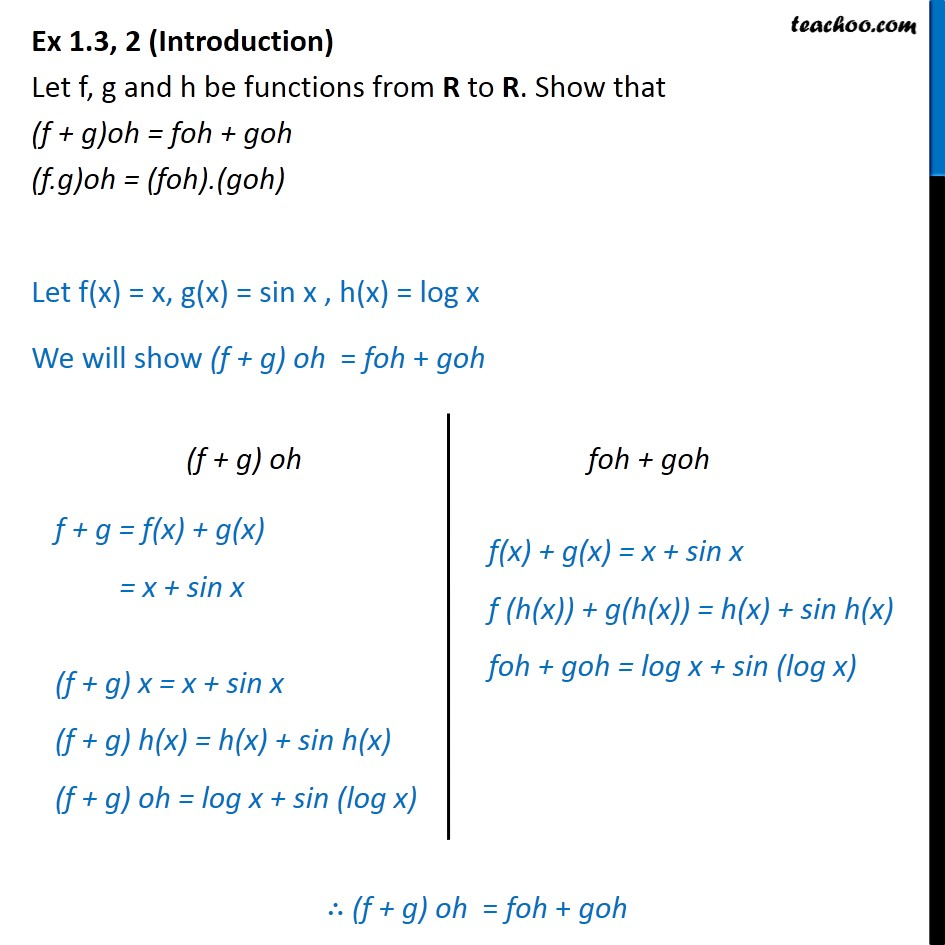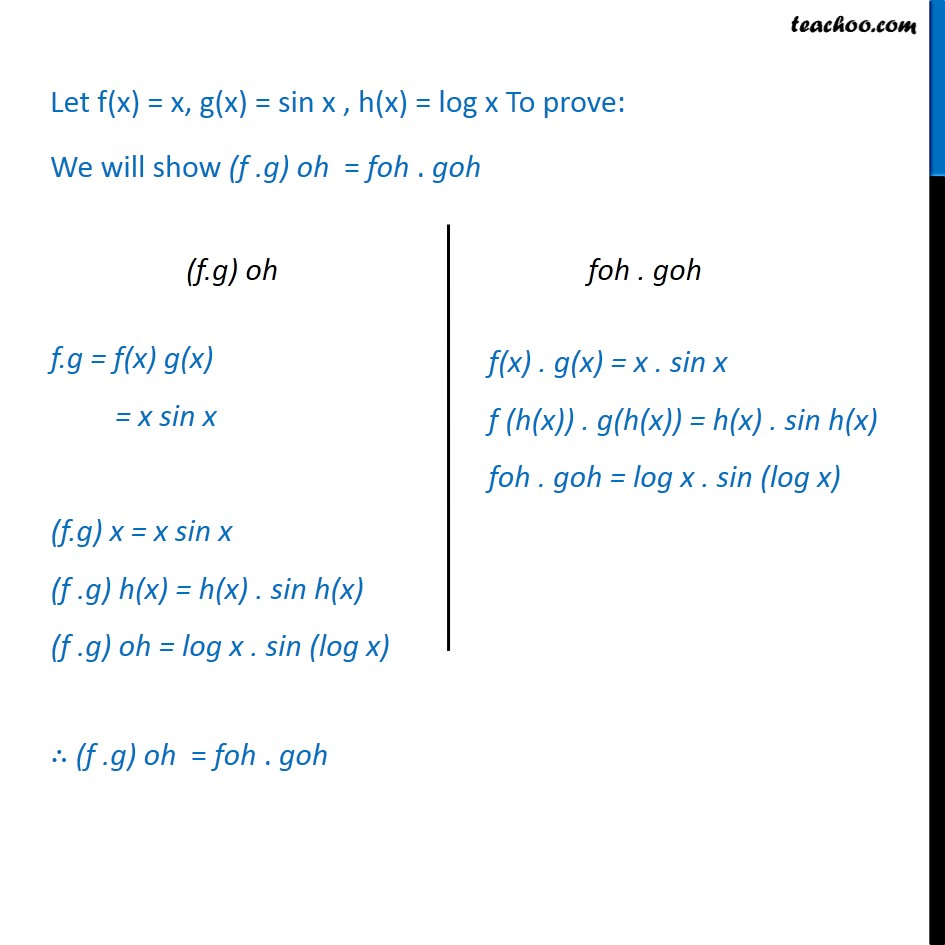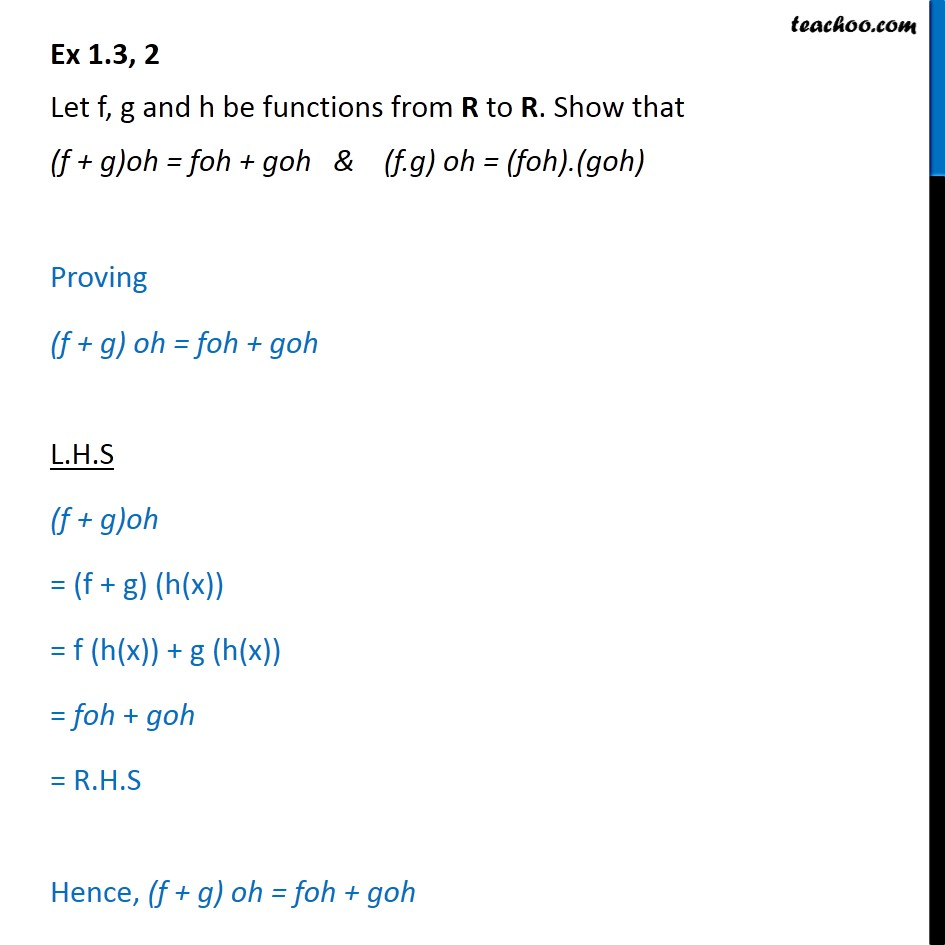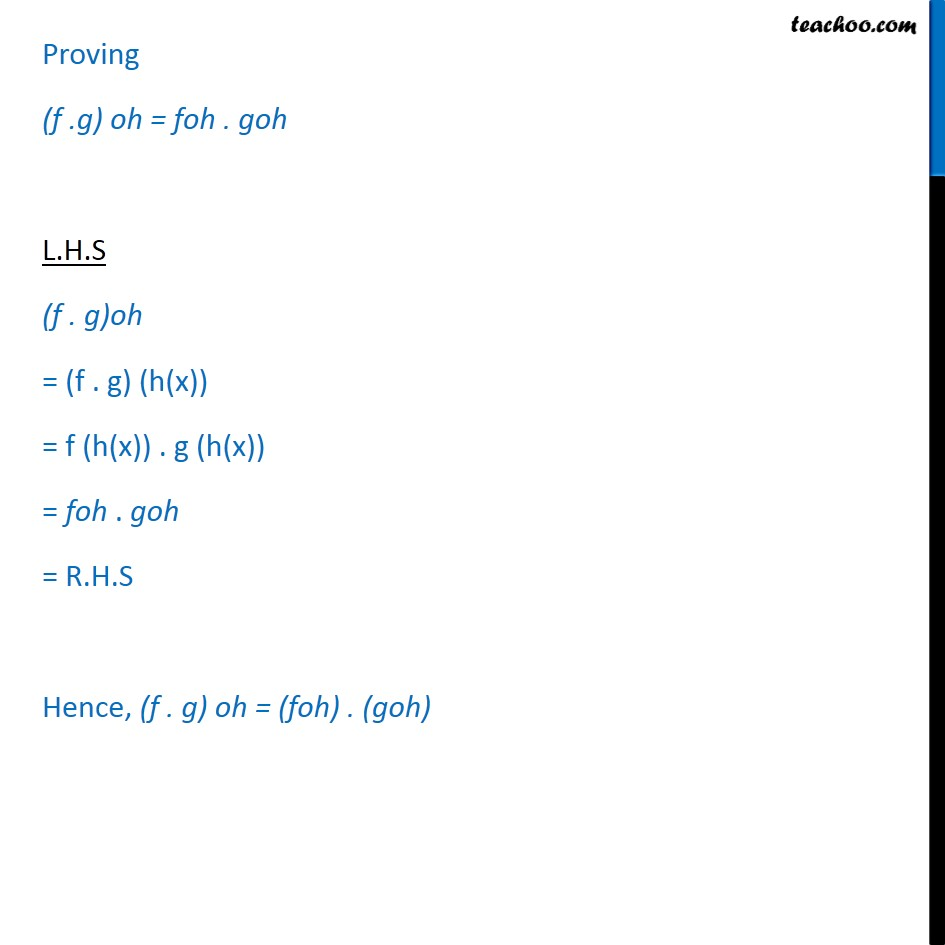Composite functions

Chapter 1 Class 12 Relation and Functions
Concept wiseIntroducing your new favourite teacher - Teachoo Black, at only ₹83 per month

### Transcript

Ex 1.3, 2 (Introduction) Let f, g and h be functions from R to R. Show that (f + g)oh = foh + goh (f.g)oh = (foh).(goh) Let f(x) = x, g(x) = sin x , h(x) = log x We will show (f + g) oh = foh + goh Let f(x) = x, g(x) = sin x , h(x) = log x To prove: We will show (f .g) oh = foh . goh (f .g) oh = foh . goh Ex 1.3, 2 Let f, g and h be functions from R to R. Show that (f + g)oh = foh + goh & (f.g) oh = (foh).(goh) Proving (f + g) oh = foh + goh L.H.S (f + g)oh = (f + g) (h(x)) = f (h(x)) + g (h(x)) = foh + goh = R.H.S Hence, (f + g) oh = foh + goh Proving (f .g) oh = foh . goh L.H.S (f . g)oh = (f . g) (h(x)) = f (h(x)) . g (h(x)) = foh . goh = R.H.S Hence, (f . g) oh = (foh) . (goh)